plot 订阅
matlab中二维线画图函数。

plot(y)等

plot

matlab
plot语法
plot函数的基本调用格式为：1、plot(y)当y为向量时，是以y的分量为纵坐标，以元素序号为横坐标，用直线依次连接数据点，绘制曲线。若y为实矩阵，则按列绘制每列对应的曲线。2、plot(x,y)若y和x为同维向量，则以x为横坐标,y为纵坐标绘制连线图。若x是向量，y是行数或列数与x长度相等的矩阵，则绘制多条不同色彩的连线图，x被作为这些曲线的共同横坐标。若x和y为同型矩阵，则以x,y对应元素分别绘制曲线，曲线条数等于矩阵列数。3、plot(x1,y1,x2,y2,……)在此格式中，每对x,y必须符合plot(x,y)中的要求，不同对之间没有影响，命令将对每一对x,y绘制曲线。以上三种格式中的x,y都可以是表达式。plot是绘制一维曲线的基本函数，但在使用此函数之前，须先定义曲线上每一点的x以及y坐标。

• ## plot

2018-10-27 23:01:37
plot用于matlab二维绘图： 句法： 1.plot(X,Y) 在Y中创建数据的二维线图，而在X中创建相应的值。 2.plot(X,Y,LineSpec) 设置线条样式、标记符号和颜色。 3.plot(X1,Y1,...,Xn,Yn)对所有线使用相同的轴绘制多个x，Y对...
plot用于matlab二维绘图：
句法：
1.plot(X,Y) 在Y中创建数据的二维线图，而在X中创建相应的值。
2.plot(X,Y,LineSpec) 设置线条样式、标记符号和颜色。
3.plot(X1,Y1,...,Xn,Yn)对所有线使用相同的轴绘制多个x，Y对。
4.plot(X1,Y1,LineSpec1,...,Xn,Yn,LineSpecn)  设置每一行的线条样式、标记类型和颜色。你可以把X，Y，L：tneSpec三重态和x，v对混合在一起
5.plot(Y) 创建Y中数据的二维线图与每个值的索引。 6.plot(Y,LineSpec)


展开全文matlab
• plot 教程plot 教程plot 教程plot 教程plot 教程plot 教程plot 教程plot 教程plot 教程
• ## matplotlib.pyplot.plot()参数详解

万次阅读 多人点赞 2018-04-03 12:17:43
在交互环境中查看帮助文档： import matplotlib.pyplot as ...help(plt.plot) 以下是对帮助文档重要部分的翻译： plot函数的一般的调用形式： #单条线： plot([x], y, [fmt], data=None, **kwargs) #多条线一...
https://matplotlib.org/api/pyplot_summary.html

在交互环境中查看帮助文档：

import matplotlib.pyplot as plt
help(plt.plot)

以下是对帮助文档重要部分的翻译：

plot函数的一般的调用形式：

#单条线：
plot([x], y, [fmt], data=None, **kwargs)
#多条线一起画
plot([x], y, [fmt], [x2], y2, [fmt2], ..., **kwargs)

可选参数[fmt] 是一个字符串来定义图的基本属性如：颜色（color），点型（marker），线型（linestyle），

具体形式  fmt = '[color][marker][line]'

fmt接收的是每个属性的单个字母缩写，例如：

plot(x, y, 'bo-')  # 蓝色圆点实线

若属性用的是全名则不能用*fmt*参数来组合赋值，应该用关键字参数对单个属性赋值如：

plot(x,y2,color='green', marker='o', linestyle='dashed', linewidth=1, markersize=6)

plot(x,y3,color='#900302',marker='+',linestyle='-')

常见的颜色参数：**Colors**

 

也可以对关键字参数color赋十六进制的RGB字符串如 color='#900302'

    =============    ===============================
character        color
=============    ===============================
'b'          blue 蓝
'g'          green 绿
'r'          red 红
'c'          cyan 蓝绿
'm'          magenta 洋红
'y'          yellow 黄
'k'          black 黑
'w'          white 白
=============    ===============================

 点型参数**Markers**,如：marker='+' 这个只有简写，英文描述不被识别

=============    ===============================
character        description
=============    ===============================
'.'          point marker
','          pixel marker
'o'          circle marker
'v'          triangle_down marker
'^'          triangle_up marker
'<'          triangle_left marker
'>'          triangle_right marker
'1'          tri_down marker
'2'          tri_up marker
'3'          tri_left marker
'4'          tri_right marker
's'          square marker
'p'          pentagon marker
'*'          star marker
'h'          hexagon1 marker
'H'          hexagon2 marker
'+'          plus marker
'x'          x marker
'D'          diamond marker
'd'          thin_diamond marker
'|'          vline marker
'_'          hline marker
=============    ===============================

线型参数**Line Styles**，linestyle='-'

    =============    ===============================
character        description
=============    ===============================
'-'          solid line style 实线
'--'         dashed line style 虚线
'-.'         dash-dot line style 点画线
':'          dotted line style 点线
=============    ===============================

样例1

函数原型：matplotlib.pyplot.plot(*args, scalex=True, scaley=True, data=None, **kwargs)
>>> plot('xlabel', 'ylabel', data=obj)
解释：All indexable objects are supported. This could e.g. be a dict, a pandas.DataFame or a structured numpy array.
data 参数接受一个对象数据类型，所有可被索引的对象都支持，如 dict 等

import matplotlib.pyplot as plt
import numpy as np
fin=open("para.txt")
a=[]
for i in fin:
a.append(float(i.strip()))
a=np.array(a)
a=a.reshape(9,3)
'''
a=np.random.random((9,3))*2 #随机生成y

y1=a[0:,0]
y2=a[0:,1]
y3=a[0:,2]

x=np.arange(1,10)

ax = plt.subplot(111)
width=10
hight=3

ax.axes.set_xlim(-0.5,width+0.2)
ax.axes.set_ylim(-0.5,hight+0.2)

plotdict = { 'dx': x, 'dy': y1 }
ax.plot('dx','dy','bD-',data=plotdict)

ax.plot(x,y2,'r^-')
ax.plot(x,y3,color='#900302',marker='*',linestyle='-')

plt.show()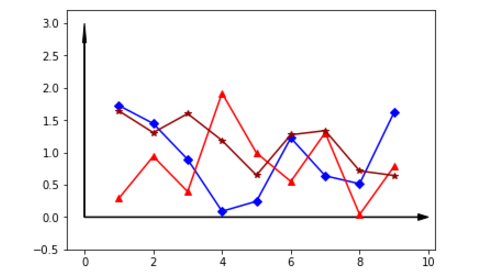样例2，

import matplotlib.pyplot as plt
import numpy as np

x = np.arange(0, 2*np.pi, 0.02)
y = np.sin(x)
y1 = np.sin(2*x)
y2 = np.sin(3*x)
ym1 = np.ma.masked_where(y1 > 0.5, y1)
ym2 = np.ma.masked_where(y2 < -0.5, y2)

lines = plt.plot(x, y, x, ym1, x, ym2, 'o')
#设置线的属性
plt.setp(lines, linewidth=1)
plt.setp(lines, linewidth=2)
plt.setp(lines, linestyle='-',marker='^',markersize=4)
#线的标签
plt.show()例3 ：圆

import numpy as np
import matplotlib.pyplot as plt

theta = np.arange(0, 2*np.pi, 0.01)
xx = [1,2,3,10,15,8]
yy = [1,-1,0,0,7,0]
rr = [7,7,3,6,9,9]

fig = plt.figure()

i = 0
while i < len(xx):
x = xx[i] + rr[i] *np.cos(theta)
x = xx[i] + rr[i] *np.cos(theta)
axes.plot(x,y)
axes.plot(xx[i], yy[i], color='#900302', marker='*')
i = i+1
width = 20
hight = 20
plt.show()


展开全文matplotlib pyplot
• ## R语言作图——density plot(密度图)

万次阅读 热门讨论 2018-10-09 13:22:57
上次分享了小提琴曲线(violin plot)的作图方法,今天小仙同学给大家介绍一下如何用R画出漂亮的密度图(density plot)。 Step1. 绘图数据的准备 首先还是要把你想要绘图的数据调整成R语言可以识别的格式excel中保存成...
原创 黄小仙
上次分享了小提琴曲线(violin plot)的作图方法,今天小仙同学给大家介绍一下如何用R画出漂亮的密度图(density plot)。
Step1. 绘图数据的准备
首先还是要把你想要绘图的数据调整成R语言可以识别的格式，把excel保存成csv格式。
数据的格式如下图：一列表示一种变量，第一行是列名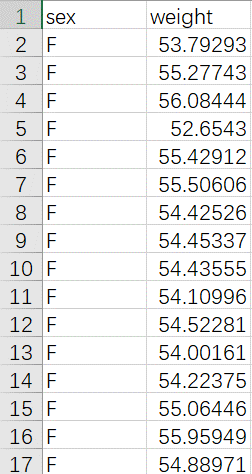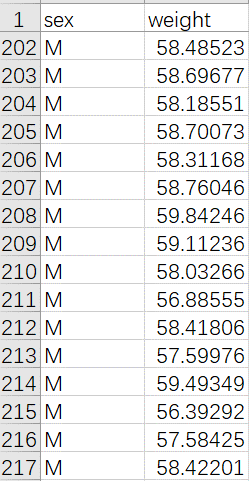Step2. 绘图数据的读取
Step3.绘图所需package的安装、调用
install.package(“ggplot2”)
#注释：ggplot2是目前公认绘图很强的一个安装包，如果已经安装，这句就不需要啦
library(ggplot2)
#注释：package使用之前需要调用(每次用前都要调用哦)
Step4绘图
p<-ggplot(data, aes(x = weight))
#注释：x轴表示weight，y轴表示频率就不需要指定啦
p + geom_density(color = “black”, fill = “gray”)
#注释：color是线条的颜色参数，fill表示填充颜色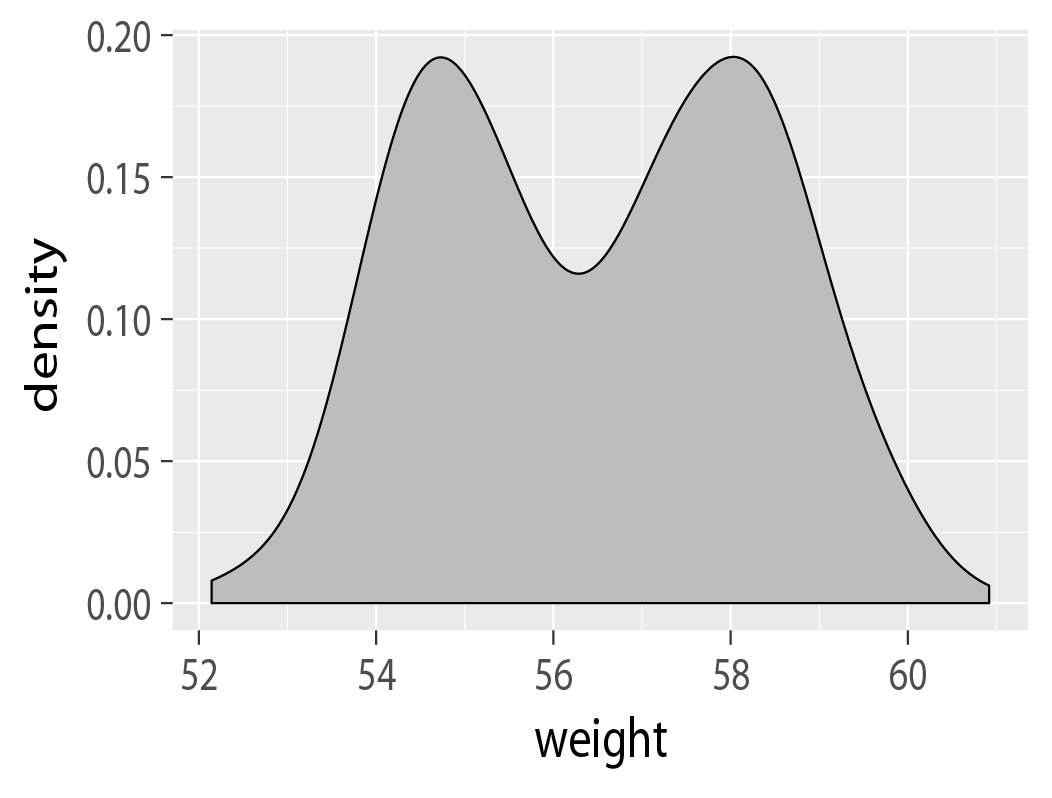Step5.美化
p + geom_density(aes(color = sex))
#注释:按照性别不同组改变线条颜色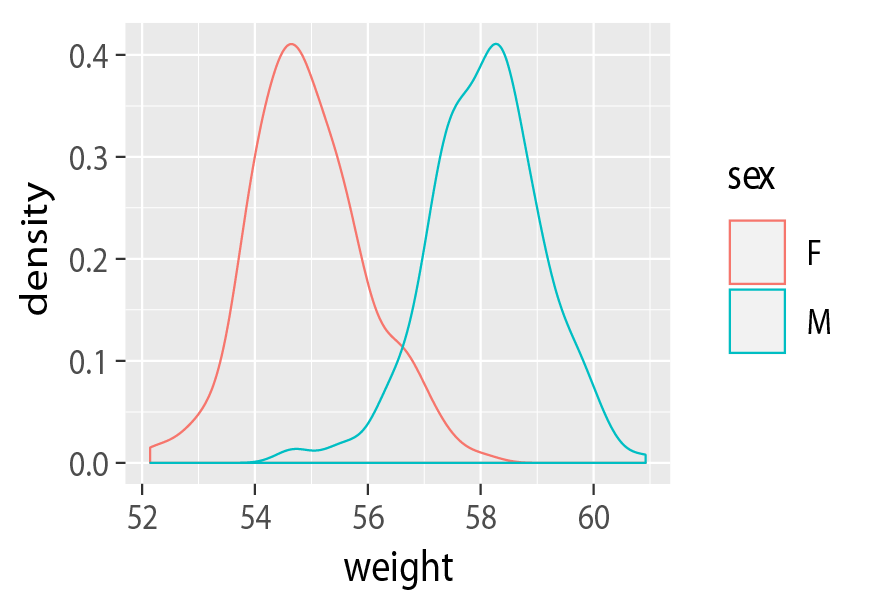p + geom_density(aes(fill = sex), alpha=0.4)
#注释:按照性别不同组改变填充颜色，alpha表示调整透明度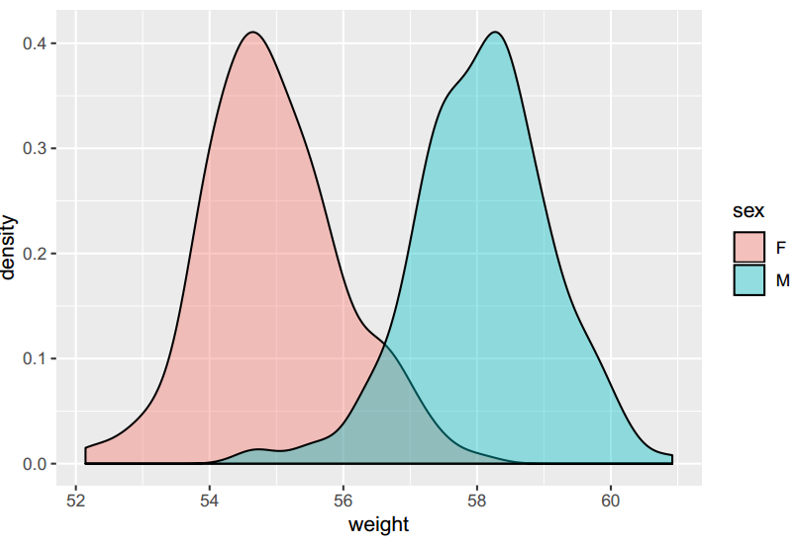到这里你已经可以画出比较高B格的density plot了，不过有些同学可能有
特殊的要求，比如把两组的平均值画出来，那本小仙再小秀一下?1.准备平均值数据并读取
2.画图
p+ geom_density(aes(color = sex), alpha=0.4)+
geom_vline(data = mean, aes(xintercept = mean, color=sex),linetype=“dashed”)
#注释:前面是和之前一样作图语句，在后面直接加上这一句就好啦，xintercept表示x轴截断线，dashed表示是虚线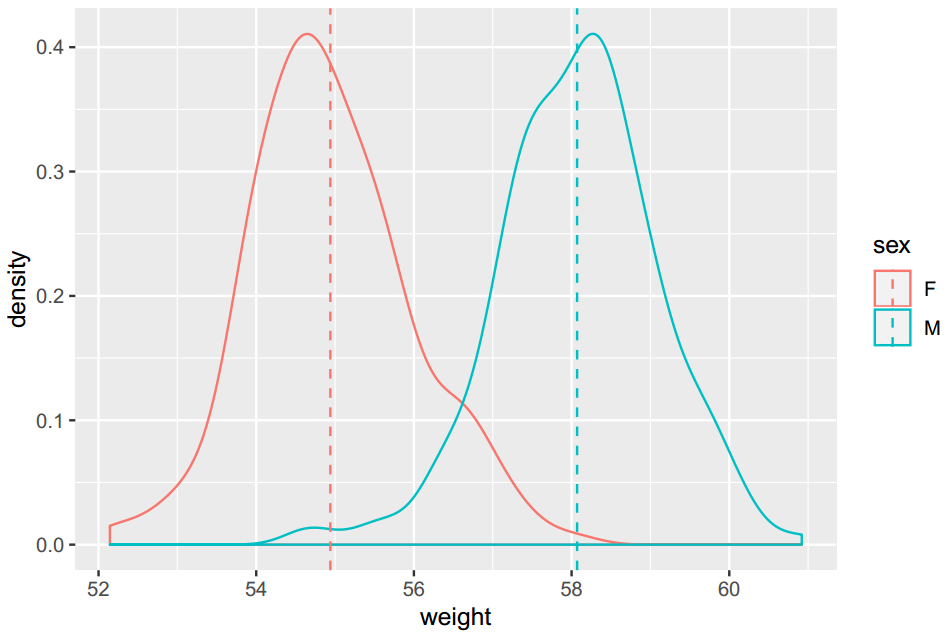好啦，今天的分享就到这里了，希望可以帮助到大家。有什么新的作图要求，可以留言给我哦！
导出高清图的方法在这里：
R语言作图技巧——导出高清图
R语言作图系列还有：
R语言作图——Histogram
R语言作图——Violin plot
R语言作图——Facet violin plot
R语言作图——Line plot
R语言作图——Density plot
R语言作图——Bubble matrix
R语言作图——Pie chart
（公众号：生信了）展开全文R语言 作图 ggplot2
• ## matlab plot函数详解

万次阅读 多人点赞 2018-04-28 17:04:08
在matlab中，plot函数用来绘制二维图像。1.plot默认格式 plot(x,y)这种格式中，若x,y是向量，则它们必须具有相同的长度。函数将以x为横轴，绘制y。 若x,y都是矩阵，则它们必须具有相同的尺寸，plot函数将针对x的各...
  在matlab中，plot函数用来绘制二维图像。1.plot默认格式  plot(x,y)这种格式中，若x,y是向量，则它们必须具有相同的长度。函数将以x为横轴，绘制y。                                   若x,y都是矩阵，则它们必须具有相同的尺寸，plot函数将针对x的各列绘制y的每列。更确切的说，将x和y的对应的各列取出来，绘制曲线。比如x和y分别为n*n的矩阵，则plot函数将x的第1列和y的第1列对应取出来，绘制一条曲线，然后将x的第二列与y的第二列对应起来，绘制一条曲线，如此下去直到第n条匹配绘制完成。                                    若x和y之一为向量，另一个为矩阵，则矩阵必须有一维与向量的长度相等。如果矩阵的行数等于向量的长度，则针对向量绘制矩阵的每列；如果矩阵的列数等于向量长度，则针对向量绘制矩阵的每行；若矩阵为方阵，则针对向量绘制矩阵的每列。                                      若x和y之一为标量，另一个为标量或者向量，则绘制离散的点；然而想要看到绘制的点，用户必须指定表示点位的记号。2.plot属性应用  带属性的格式中。增加了线属性的设置，这些属性包括线型设置，绘制线条时是否使用标记符号以及使用什么样的标记符号，线条的颜色、粗细等等。  在plot函数中，无论是线型、标记符号还是颜色，具体制定时，都是以字符串的形式出现。字符串中的颜色可以以任何顺序出现，用户也可以省略其中的一个或多个选项。如果用户省略了线条样式，但制定了标记字符，则plot函数只显示标记符，但不会显示线条。  plot函数在绘制线条时，线条属性主要包括三个方面，一是线型的使用。符号含义符号含义符号含义符号含义-细实线：虚点线-.点画线--虚画线  二是颜色的使用，其中最常用的颜色如下所示，更多的颜色可以采用RGB来表示。点线颜色允许的设置值符号含义符号含义符号含义符号含义r红b蓝y黄w白g绿c青k黑m品红  三是标志符的应用，它可以更有效的显示出数据点的位置。标识符符号与含义符号含义符号含义符号含义符号含义.实心点<朝左三角d菱形p五角星+十字号>朝右三角h六角星s方块*星号v朝下三角o空心圆圈x叉字符^朝上三角        matlab对plot函数中线条属性的修改，提供了属性名称和属性值的设置方法。线条属性名称和设置值属性名称含义属性值备注Color设置线条或点的颜色[r,g,b],各颜色强度介于0~1常用颜色可使用色彩符号进行设置使用RGB颜色数据也可默认蓝色LineStyle线型细实线、虚点线、点画线、虚画线通过线型符号设置默认细实线LineWidth线条宽度/粗细正实数正版出版物使用1.4为宜默认为0.5Marker数据点标识符菱形、空心圆圈等13种通过标识符符号设置MarkerSize标识符大小正实数默认值为6.0MarkerEdgeColor标识符边界色彩[r,g,b],各颜色强度介于0~1 MarkerFaceColor标识符内部填充色彩[r,g,b],各颜色强度介于0~1 3.其他几种格式plot(x1,y1,...,xn,yn)       在这种格式中，将使用相同的坐标轴绘制多条曲线。plot(x1,y1,linespec1,...,xn,yn,linespecn)       这种格式允许用户对每条线进行属性设置plot(y)       这种格式中，只有数据y，plot将绘制二维的线条。具体来讲，针对y的每个数据，以数据的索引当做x与其值配对绘制曲线。如果y是向量，那么x轴的尺度范围从1到y的长度。如果y是矩阵，则绘制y的每列，列中数据对应的x，则取各值对应的行号。若y是复数，则复数的实部设定为x，虚部设定为y。ploy(y,linespec)       统一设定各线条的属性。plot(ax,______)       这种格式不是在当前的轴框绘图，而是在由句柄ax指定的轴框内绘图，这种格式允许用户对特定绘图对象进行属性设置。h=plot(______)      这种格式返回由图中各线条的句柄构成的列向量h，即h中的每个元素就是图中一条线的句柄，当绘制多条线时，用户可通过某条线的句柄对该线进行特定的修改。
展开全文• <div><pre> --------------------------- Assertion Failed: Abort=Quit, Retry=Debug, Ignore=Continue --------------------------- Exception in RHost run loop: Microsoft.R.Host.Client....plot...
• 一、用默认设置绘制折线图 import matplotlib.pyplot as plt x_values=list(range(11)) #x轴的数字是0到10这11个整数 y_values=[x**2 for x in x_values] ...#用plot函数绘制折线图，线条颜色设置为绿...Python matplotlib
• ## plt.plot()的使用方法以及参数介绍

万次阅读 多人点赞 2019-05-21 09:07:48
偶然的一次操作，对plt.plot()中，仅仅传入了一个列表，然后逻辑就和预想的出现了偏差。 plt.plot() plt.plot() 参数介绍： x, y : array-like or scalar The horizontal / vertical coordinates of the data ...matplotlib python pyplot
• ## MATLAB中plot函数的用法

万次阅读 多人点赞 2018-04-23 15:47:04
使用plot绘制二维图像本文转自http://blog.sina.com.cn/s/blog_d8f783c90102woqb.html以及...本文仅介绍plot函数的基本用法——使用plot函数绘制二维点图和线...matlab
• 在Matlab使用Plot函数实现数据动态显示方法总结中介绍了两种实现即时数据动态显示的方法。考虑到使用python的人群日益增多，再加上本人最近想使用python动态显示即时的数据，网上方法很少，固总结于此。 示例代码...python 函数 数据
• plot 例子plot 例子plot 例子plot 例子plot 例子plot 例子plot 例子
• import matplotlib.pyplot as plt from numpy.random import randn plt.style.use('ggplot') plot_data1=randn(50).cumsum() plot_data2=randn(50).cumsum() plot_data3=randn(50).cumsum...plot_data4=randn(50).c...matplotlib
• ## plot3d

2019-03-09 10:30:00
plot3d plot3Da format for structured grid data that was popularized by NASA's CFD visualization Author: kemina Emacs 24.5.1 (Org mode 8.2.10) 转载于:https://www.cnbl...
• ## plt.plot

千次阅读 2018-08-30 15:10:38
• 调用签名：plt.plot(x, y, ls="-", lw=2, label=“plot figure”) x: x轴上的数值 y: y轴上的数值 ls：折线图的线条风格 lw：折线图的线条宽度 label：标记图内容的标签文本 matplotlib.pyplot import matplotlib....
• 转自：https://blog.csdn.net/jirryzhang/article/details/77374702 MATLAB plot画线的颜色设定 plot中画线的颜色通常是八种： 标记符 颜色 r 红 g 绿 b 蓝 c 蓝绿 m 紫红 y ...
• 指定颜色、线型与宽度、横纵坐标数值与标签大小 clo={‘k-’,‘k:’,‘k-.’,‘k–’,‘k-’};...plot(h1(i).h,c,‘LineWidth’, str2num(lin(i))); hold on; end title(‘EWHM,z=0.8’,‘Font...
• plot为高级绘图函数，实际调用的实地基函数line。 line函数在后面章节会详细介绍。 1. plot(Y) 若Y为向量，产生向量Y对应于其索引值的曲线；若Y为矩阵，生成矩阵的每列对应于行数的曲线集合；若Y为负数，等价于...matlab figure
• ## pandas plot参数

万次阅读 2018-09-22 10:50:47
封装matplotlib的plot函数 pandas.plot DataFrame.plot(x=None, y=None, kind=‘line’, ax=None, subplots=False, sharex=None, sharey=False, layout=None, figsize=None, use_index=True, title=None, grid=...python pandas
• ## 箱形图box plot 和 小提琴图violin plot

万次阅读 多人点赞 2018-02-02 10:24:37
一、箱形图box plot 1）箱型图概念 箱形图（Box-plot）又称为盒须图、盒式图或箱线图，是一种用作显示一组数据分散情况资料的统计图。因形状如箱子而得名。 箱形图于1977年由美国著名统计学家约翰·图基（John ...
• 深度学习采用数据驱动（data driven)的方法进行现实...为了走近这些数据，plot data是一个方法。import matplotlib.pyplot as pltplt.scatter(x,y,s=50,c="green") # 散点图plt.hist(x,30) # 直方图 ...
• 使用DataFrame的plot方法绘制图像会按照数据的每一列绘制一条曲线，默认按照列columns的名称在适当的位置展示图例，比matplotlib绘制节省时间，且DataFrame格式的数据更规范，方便向量化及计算。 二、df.plot() ...
• ## Matlab plot

千次阅读 2010-05-21 14:43:00
二维曲线绘图语法plot(Y)plot(X1,Y1,...)plot(X1,Y1,LineSpec,...)plot(...,PropertyName,PropertyValue,...)plot(axes_handle,...)h = plot(...)hlines = plot(v6,...)描述plot(Y)如果Y是m×n的数组，以1:m为X横...matlab c
• plot 函数的语法plot([x], y, [fmt], **kwargs)3. 一张图画好几条线 1. 加载宏包 使用 matplotlib 包画图时，我们一般加载里面的 pyplot，并命名为 plt，然后使用 plot 函数画图。 # 导入 matplotlib 中的 plot， ...python matplotlib pyplot
• ## plot参数详解

千次阅读 2019-03-09 15:36:23
封装matplotlib的plot函数 pandas.plot DataFrame.plot(x=None, y=None, kind=‘line’, ax=None, subplots=False, sharex=None, sharey=False, layout=None, figsize=None, use_index=True, tit...matplotlib
• ## Box Plot

千次阅读 2015-08-03 07:35:19
一个典型的Box Plot是基于以下五个值计算而来的a. 一组样本的最小值b. 一组样本的最大值c. 一组样本的中值d. 下四分位数（Lower Quartile / Q1）e. 上四分位数（Upper Quartile / Q3）根据这五个值构建出来基本的Box...机器学习 box
• 使用该类的plot函数，如果只给该函数一个列表值，则列表值即为y值，横坐标值默认为列表值的索引值；如果给该属性两个具有相同长度的列表值，则前一个列表值为y值，后一个列表值为对应的x值。使用类的函数ylabel可以...
• 本文接下来要讲解一下Matlab中最基本的Plot的用法 Plot的定义和用法 此 MATLAB 函数 创建 Y 中数据对 X 中对应值的二维线图。 如果 X 和 Y 都是向量，则它们的长度必须相同。plot 函数绘制 Y 对 X 的图。 如果 X ...Matlab...